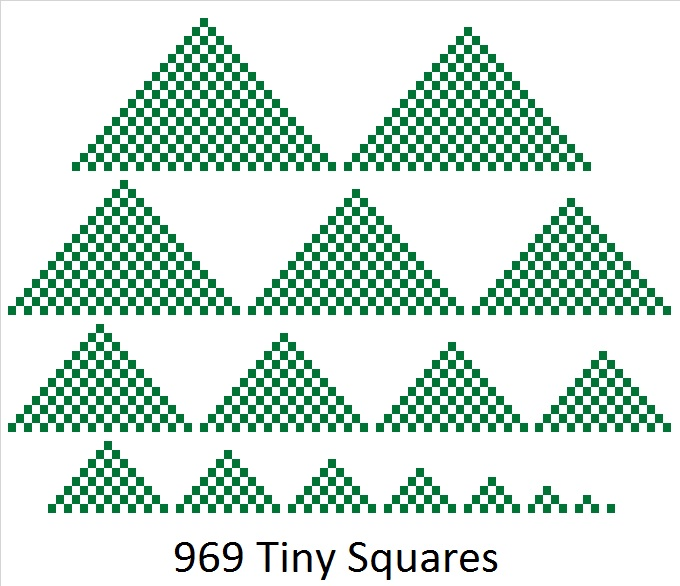# 969 is the 17th Tetrahedral Number and the 17th Nonagonal Number

A tetrahedron is a pyramid whose base and sides are all triangles.

The nth tetrahedral number is the sum of the first n triangular numbers. So if you made a pyramid of the first n triangular numbers, you would get the nth triangular pyramidal number, also known as the nth tetrahedral number.

969 is the 17th tetrahedral number.That image might look a little like a Christmas tree lot where you could select a tree in several different sizes. If we had tiny cubes instead of squares, we could stack them on top of each other to make a tetrahedron. That is the visual reason why 969 is a tetrahedron.

Look at the graphic below of a portion of Pascal’s triangle. You can easily see the first 19 counting numbers. The first 18 triangular numbers are highlighted in red, and the first 17 tetrahedral numbers are highlighted in green. The 16th tetrahedral number, 816, plus the 17th triangular number, 153, equals 969.Because of its spot on Pascal’s triangle, I know that (17·18·19)/(1·2·3) = 969. That is the algebraic reason 969 is a tetrahedral number.

969 is also the 17th nonagonal number because 17(7·17 – 5)/2 = 969. I am not going to try to illustrate a 9-sided figure, but I’m sure it would be a cool image if I could.

All of this means that 969 is the 17th tetrahedral number AND the 17th nonagonal number. 1 is the smallest number to be both a tetrahedral number and a nonagonal number. 969 is the next smallest number to be both. Amazingly, it is the 17th of both, too!

969 obviously is a palindrome in base 10.

In base 20, it is 289. I find that quite curious because 17² = 289, and 17 is a factor of 969. Why would we write this number as 289 in base 20? Because 2(20²) + 8(20) + 9(1) = 969

Because 17 is one of its factors, 969 is the hypotenuse of a Pythagorean triple:
456-855-969 which is 57 times (8-15-17)

• 969 is a composite number.
• Prime factorization: 969 = 3 × 17 × 19
• The exponents in the prime factorization are 1, 1, and 1. Adding one to each and multiplying we get (1 + 1)(1 + 1)(1 + 1) = 2 × 2 × 2 = 8. Therefore 969 has exactly 8 factors.
• Factors of 969: 1, 3, 17, 19, 51, 57, 323, 969
• Factor pairs: 969 = 1 × 969, 3 × 323, 17 × 57, or 19 × 51
• 969 has no square factors that allow its square root to be simplified. √969 ≈ 31.12876This site uses Akismet to reduce spam. Learn how your comment data is processed.